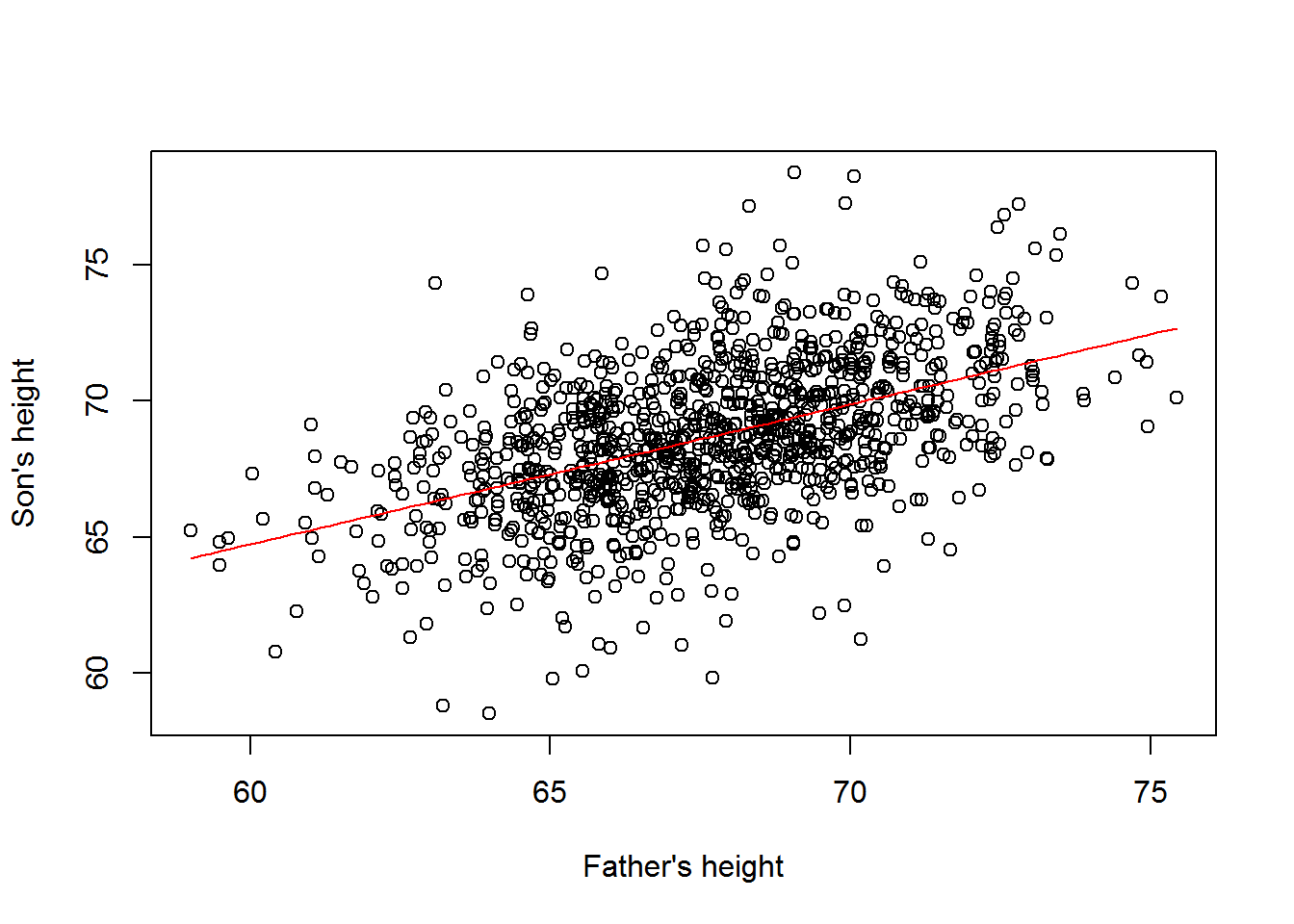### Representation of linear Models

• The general forumula for a linear model is $$Y_i = \beta_0 + \beta_1 x_{1i} + ... + \beta_j x_{ji}+ \varepsilon$$
• $$Y_i$$ is each actual data point to be fit in the model
• $$\beta_0$$ is the a constant, base value from which all data is relative to (graphically it is the intercept)
• $$x_{ji}$$ is each variable to be included in the model
• Each $$i$$ corresponds to one value in data $$Y$$, $$1,...,N$$
• Each $$j$$ corresponds to on variable in the model, the number of variables depending on the models design, $$1,..,M$$
• $$\beta_j$$ is the co-variate or coefficient modifying each variable to result in the fit of the model
• $$\varepsilon$$ is the residual difference between the model and each individual data point
• In matrix notation: $\left(\begin{array}{c}Y_1\\Y_2\\\vdots\\Y_N\end{array}\right) = \left(\begin{array}{cccc}1 & x_{1,1} & x_{1,2} & x_{1,...} & x_{1,M}\\1 & x_{2,1} & x_{2,2} & x_{2,...} & x_{2,M}\\\vdots & \vdots & \vdots & \vdots & \vdots\\1 & x_{N,1} & x_{N,2} & x_{N,...} & x_{N,M}\end{array}\right)\left(\begin{array}{c}\beta_0\\\beta_1\\\beta_2\\\vdots\\\beta_M\end{array}\right) + \left(\begin{array}{c}\varepsilon_1\\\varepsilon_2\\\vdots\\\varepsilon_N\end{array}\right)$
• $$Y_1,..,Y_N$$ represents each point of data
• The first column of 1s in the $$x$$ matrix is there because values in that column will always be matrix multiplied by the value of $$\beta_0$$. Since $$\beta_0$$ is a constant, it should not change according to any data.
• Each remaining column in the $$X$$ matrix represents a variable of interest in the model from 1 to $$M$$
• Each row in the $$X$$ matrix represents the variables applied to each point of data, 1 through $$N$$, in $$Y$$
• The one column $$\beta$$ matrix is what is to be calculated by the linear model
• When completed, each “row” of the model will completely calculate each point in $$Y$$
• Model can be more simply denoted by: $Y = X\beta + \varepsilon$

### Solving linear models by least squares

• The least squares regression is the model that minimizes the square of the residuals (i.e. the difference between an actual value and the expected value based on the model, at the same point)
• The sum of squares can ordinarly be writted as $$\sum_{i=1}^N R_i^2$$, where $$R$$ is the residual
• In matrix notation this would be represented as $$(Y-X\beta)^T(Y-X\beta)$$
• $$X\beta$$ represents the dot product of all variables and their co-variates for each data point, thus representing a vector of all expected values
• $$Y$$ represents the vector of all actual values
• Therefore $$(Y-X\beta)$$ is the vector of all residuals
• Finally, recall the crossproduct of a single matrix is the way to obtain the square of that matrix
• The first derivative of an equation allows us to determine minimums (and maximums) based on where the derivative is equal to zero
• The derivative of the matrix-form residual equation equal to zero (to find the minumum) is $$2X^T(Y-X\hat{\beta}) = 0$$
• Formula is similar to the derivative of $$f(x)^2$$ being $$2f(x)f\prime(x)$$
• $$\hat{\beta}$$ because $$\beta$$ is being calculated
• Solving for zero results in $$2X^T(Y-X\hat{\beta})\Rightarrow X^TX\hat{\beta} = X^TY \Rightarrow \hat{\beta} = (X^TX)^{-1}X^TY$$
• Remember that dividing by a matrix requires instead multiplying by that matrix’s inverse (see last step)
• Notice that the final equation contains a two cross products
• Thus, the equation to solve a linear model is: $\hat{\beta} = (X^TX)^{-1}X^TY$

# Linear model R example

• Finding the regression line between father vs. son height
library(UsingR)
x=father.son$fheight y=father.son$sheight
X <- cbind(1,x)
beta <- solve(t(X)%*%X)%*%t(X)%*%y
###or, because of the cross products
beta <- solve(crossprod(X))%*%crossprod(X,y)
beta
##        [,1]
##   33.886604
## x  0.514093
• Thus, the least square line is $$f(x) = 33.89 + 0.51x$$, which looks like:• In R, the lm function is used to automatically calculate such models
fit <- lm(y ~ x)
summary(fit)
##
## Call:
## lm(formula = y ~ x)
##
## Residuals:
##     Min      1Q  Median      3Q     Max
## -8.8772 -1.5144 -0.0079  1.6285  8.9685
##
## Coefficients:
##             Estimate Std. Error t value Pr(>|t|)
## (Intercept) 33.88660    1.83235   18.49   <2e-16 ***
## x            0.51409    0.02705   19.01   <2e-16 ***
## ---
## Signif. codes:  0 '***' 0.001 '**' 0.01 '*' 0.05 '.' 0.1 ' ' 1
##
## Residual standard error: 2.437 on 1076 degrees of freedom
## Multiple R-squared:  0.2513, Adjusted R-squared:  0.2506
## F-statistic: 361.2 on 1 and 1076 DF,  p-value: < 2.2e-16

*Notice that the Estimate for the (Intercept) and x are quivalent to the beta vector calculated manually